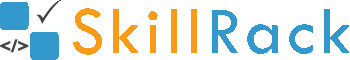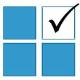ProgramID- 9229SkillRack
Print Two Digit Numbers

The program must accept N integers as the input. The program must print all the two digit integers among the N integers in reverse order as the output. If there is no two digit integer then the program must print -1 as the output.

Boundary Condition(s):
1 <= N <= 100
1 <= Each integer value <= 10^6

Example Input/Output 1:
Input:
6
10 987 75 1011 5 99

Output:
99 75 10

Example Input/Output 2:
8
203 540 897 1 6 501 150 2

Output:
-1

Max Execution Time Limit: 1000 millisecs